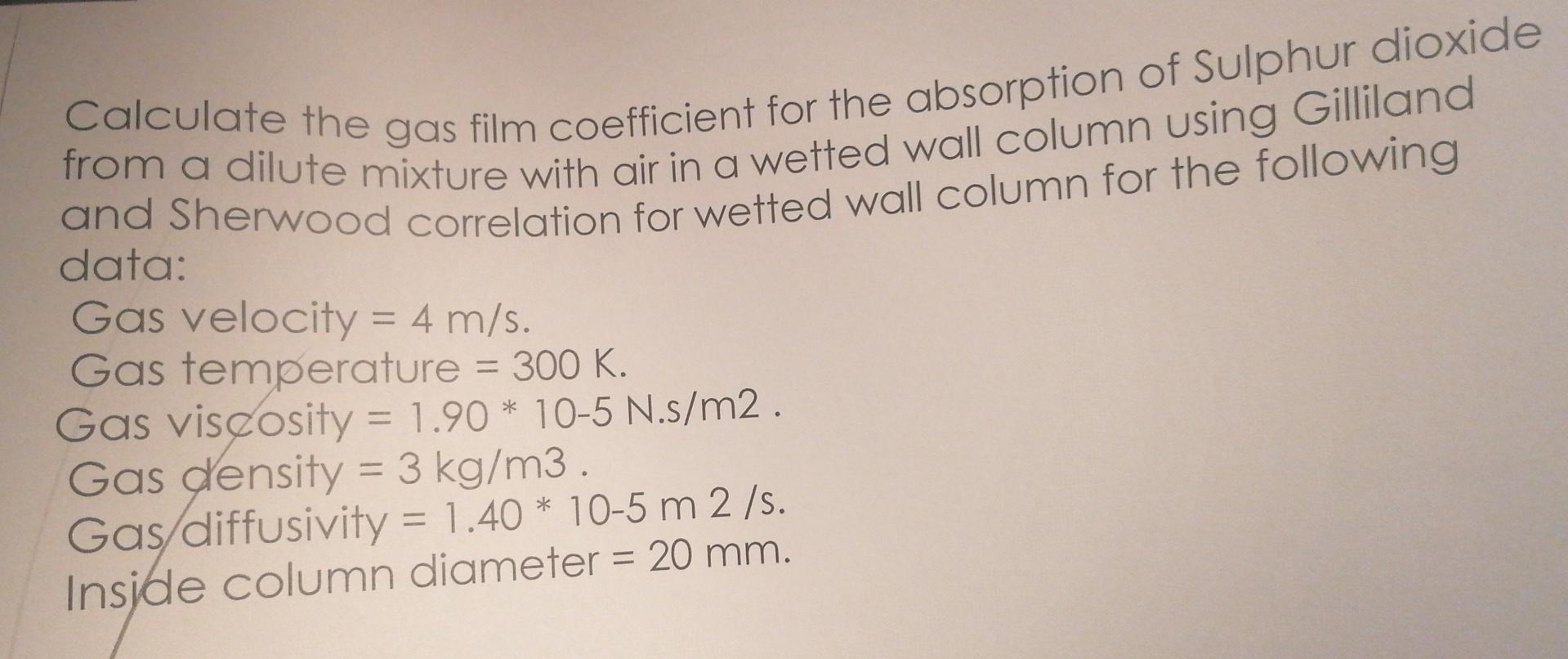# Question Solved1 AnswerCalculate the gas film coefficient for the absorption of Sulphur dioxide from a dilute mixture with air in a wetted wall column using Gilliland and Sherwood correlation for wetted wall column for the following data: Gas velocity $$=4 \mathrm{~m} / \mathrm{s}$$. Gas temperature $$=300 \mathrm{~K}$$. Gas viscosity $$=1.90 * 10-5 \mathrm{~N} . \mathrm{s} / Calculate the gas film coefficient for the absorption of Sulphur dioxide from a dilute mixture with air in a wetted wall column using Gilliland and Sherwood correlation for wetted wall column for the following data: Gas velocity \( =4 \mathrm{~m} / \mathrm{s}$$. Gas temperature $$=300 \mathrm{~K}$$. Gas viscosity $$=1.90 * 10-5 \mathrm{~N} . \mathrm{s} / \mathrm{m} 2$$. Gas density $$=3 \mathrm{~kg} / \mathrm{m} 3$$. Gas/diffusivity $$=1.40 * 10-5 \mathrm{~m} 2 / \mathrm{s}$$. Inside column diameter $$=20 \mathrm{~mm}$$.UCLXBF The Asker · Chemical EngineeringTranscribed Image Text: Calculate the gas film coefficient for the absorption of Sulphur dioxide from a dilute mixture with air in a wetted wall column using Gilliland and Sherwood correlation for wetted wall column for the following data: Gas velocity $$=4 \mathrm{~m} / \mathrm{s}$$. Gas temperature $$=300 \mathrm{~K}$$. Gas viscosity $$=1.90 * 10-5 \mathrm{~N} . \mathrm{s} / \mathrm{m} 2$$. Gas density $$=3 \mathrm{~kg} / \mathrm{m} 3$$. Gas/diffusivity $$=1.40 * 10-5 \mathrm{~m} 2 / \mathrm{s}$$. Inside column diameter $$=20 \mathrm{~mm}$$.
More
Transcribed Image Text: Calculate the gas film coefficient for the absorption of Sulphur dioxide from a dilute mixture with air in a wetted wall column using Gilliland and Sherwood correlation for wetted wall column for the following data: Gas velocity $$=4 \mathrm{~m} / \mathrm{s}$$. Gas temperature $$=300 \mathrm{~K}$$. Gas viscosity $$=1.90 * 10-5 \mathrm{~N} . \mathrm{s} / \mathrm{m} 2$$. Gas density $$=3 \mathrm{~kg} / \mathrm{m} 3$$. Gas/diffusivity $$=1.40 * 10-5 \mathrm{~m} 2 / \mathrm{s}$$. Inside column diameter $$=20 \mathrm{~mm}$$.